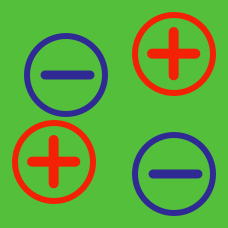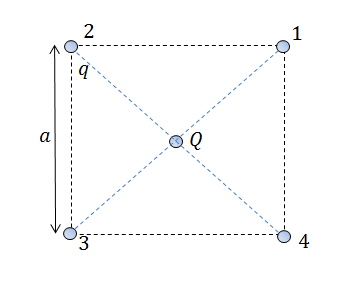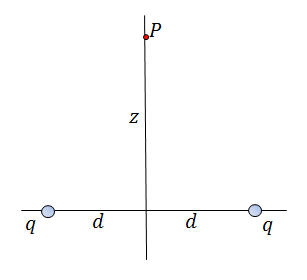Electricity and Magnetism

# Coulomb's lawFour equal charges, $q = 6 \times 10^{-6} \text{ C }$ , are situated at the corners of a square as shown in the above diagram. What is the net force (in Newtons) on the test charge $Q = 9 \times 10^{-6} \text{ C }$ at the center?

Let $\pi \approx 3$ and the permitivity of free space $\epsilon_0 \approx 9\times 10^{-12} \frac{\text{C}^2}{\text{N*m}^2}$

Two charges Q=+1 mC are placed along the x-axis at x=-2 meters and x=2 meters. The charges are fixed in space. A third charge, which is free to move and has charge q=-0.1 mC and mass m=1g, is placed along the y-axis at y=0.01 meters and released. How long does it take in seconds for the charge to come back to y=0.01?

Details and assumptions

• $k=(4 \pi \epsilon_0)^{-1}= 9 \times 10^9~Nm^2/C^2$If the magnitude of the electric field a distance $z = 9 \text{ m}$ above the midpoint between two equal charges, $q = 25 \times 10^{-12} \text{ C }$, a distance $2d =24 \text{ m}$ apart can be expressed as $E = \frac{a}{b} \text{ N/C},$ where $a$ and $b$ are coprime positive integers, what is the value of $a+b?$

Let $\pi \approx 3$ and the permitivity of free space $\epsilon_0 \approx 9\times 10^{-12} \frac{\text{C}^2}{\text{N*m}^2}$

A test charge $Q = 5 \times 10^{-6} \text{ C }$ is placed by a single point charge $q = 7 \times 10^{-6} \text{ C }$ which is at rest at a distance of $r = 4 \text{ m }$ away. If the force can be expressed as $F = \frac{a}{b} \text{ N},$ where $a$ and $b$ are coprime positive integers, what is the value of $a+b?$

Let $\pi \approx 3$ and the permitivity of free space $\epsilon_0 \approx 9\times 10^{-12} \frac{\text{C}^2}{\text{N*m}^2}$

If the magnitude of the electric field at a distance of $r = 3 \text{ m}$ from the point charge $Q = 9 \times 10^{-12} \text{ C }$ can be expressed as $E = \frac{a}{b} \text{ N/C},$ where $a$ and $b$ are coprime positive integers, what is the value of $a+b?$

Let $\pi \approx 3$ and the permitivity of free space $\epsilon_0 \approx 9\times 10^{-12} \frac{\text{C}^2}{\text{N*m}^2}$

×